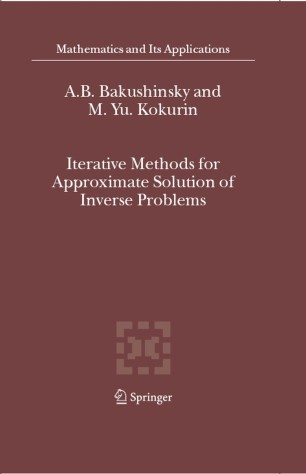# Iterative Methods for Approximate Solution of Inverse Problems

• A. B. Bakushinsky
• M. Yu. KokurinBook

Part of the Mathematics and Its Applications book series (MAIA, volume 577)

1. Front Matter
Pages i-xv
2. Pages 17-87
3. Pages 159-223
4. Pages 225-273
5. Back Matter
Pages 275-291

### Introduction

This volume presents a unified approach to constructing iterative methods for solving irregular operator equations and provides rigorous theoretical analysis for several classes of these methods. The analysis of methods includes convergence theorems as well as necessary and sufficient conditions for their convergence at a given rate. The principal groups of methods studied in the book are iterative processes based on the technique of universal linear approximations, stable gradient-type processes, and methods of stable continuous approximations. Compared to existing monographs and textbooks on ill-posed problems, the main distinguishing feature of the presented approach is that it doesn’t require any structural conditions on equations under consideration, except for standard smoothness conditions. This allows to obtain in a uniform style stable iterative methods applicable to wide classes of nonlinear inverse problems. Practical efficiency of suggested algorithms is illustrated in application to inverse problems of potential theory and acoustic scattering.

The volume can be read by anyone with a basic knowledge of functional analysis.

The book will be of interest to applied mathematicians and specialists in mathematical modeling and inverse problems.

### Keywords

brandonwiskunde algorithms functional functional analysis Mathematica mathematical modeling modeling operator

#### Authors and affiliations

• A. B. Bakushinsky
• 1
• M. Yu. Kokurin
• 2
2. 2.Mary State UniversityRussia

### Bibliographic information

• DOI https://doi.org/10.1007/978-1-4020-3122-9